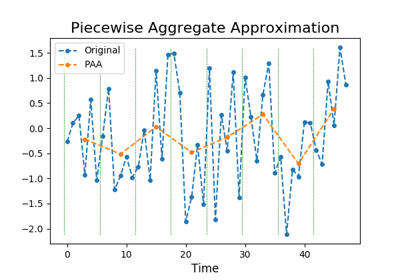# `pyts.approximation`.PiecewiseAggregateApproximation¶

class `pyts.approximation.``PiecewiseAggregateApproximation`(window_size=1, output_size=None, overlapping=True)[source]

Piecewise Aggregate Approximation.

Parameters: window_size : int, float or None (default = 1) Length of the sliding window. If float, it represents a percentage of the size of each time series and must be between 0 and 1. output_size : int, float or None (default = None) Size of the returned time series. If float, it represents a percentage of the size of each time series and must be between 0. and 1. Ignored if `window_size` is not None. It can’t be None if `window_size` is None. If you want to use `output_size` over `window_size`, you must set `window_size=None`. overlapping : bool (default = True) When `window_size=None`, `output_size` is used to derive the window size; the window size is fixed if `overlapping=True` and may vary if `overlapping=False`. Ignored if `window_size` is specified.

References

 [R5f91eb1fc1e8-1] E. Keogh, K. Chakrabarti, M. Pazzani, and S. Mehrotra, “Dimensionality reduction for fast similarity search in large time series databases”. Knowledge and information Systems, 3(3), 263-286 (2001).

Examples

```>>> from pyts.approximation import PiecewiseAggregateApproximation
>>> X = [[0, 4, 2, 1, 7, 6, 3, 5],
...      [2, 5, 4, 5, 3, 4, 2, 3]]
>>> transformer = PiecewiseAggregateApproximation(window_size=2)
>>> transformer.transform(X)
array([[2. , 1.5, 6.5, 4. ],
[3.5, 4.5, 3.5, 2.5]])
```

Methods

 `__init__`(self[, window_size, output_size, …]) Initialize self. `fit`(self[, X, y]) Pass. `fit_transform`(self, X[, y]) Fit to data, then transform it. `get_params`(self[, deep]) Get parameters for this estimator. `set_params`(self, \*\*params) Set the parameters of this estimator. `transform`(self, X) Reduce the dimensionality of each time series.
`__init__`(self, window_size=1, output_size=None, overlapping=True)[source]

Initialize self. See help(type(self)) for accurate signature.

`fit`(self, X=None, y=None)[source]

Pass.

Parameters: X Ignored y Ignored self object
`fit_transform`(self, X, y=None, **fit_params)

Fit to data, then transform it.

Fits transformer to X and y with optional parameters fit_params and returns a transformed version of X.

Parameters: X : numpy array of shape [n_samples, n_features] Training set. y : numpy array of shape [n_samples] Target values. **fit_params : dict Additional fit parameters. X_new : numpy array of shape [n_samples, n_features_new] Transformed array.
`get_params`(self, deep=True)

Get parameters for this estimator.

Parameters: deep : bool, default=True If True, will return the parameters for this estimator and contained subobjects that are estimators. params : mapping of string to any Parameter names mapped to their values.
`set_params`(self, **params)

Set the parameters of this estimator.

The method works on simple estimators as well as on nested objects (such as pipelines). The latter have parameters of the form `<component>__<parameter>` so that it’s possible to update each component of a nested object.

Parameters: **params : dict Estimator parameters. self : object Estimator instance.
`transform`(self, X)[source]

Reduce the dimensionality of each time series.

Parameters: X : array-like, shape = (n_samples, n_timestamps) X_new : array, shape = (n_samples, n_timestamps_new)

## Examples using `pyts.approximation.PiecewiseAggregateApproximation`¶Piecewise Aggregate Approximation